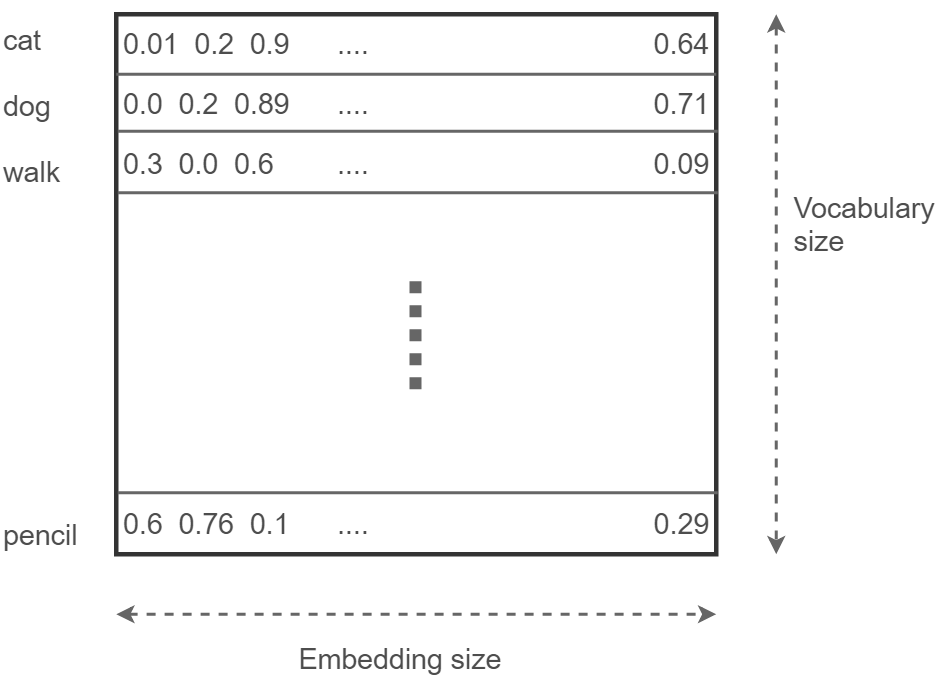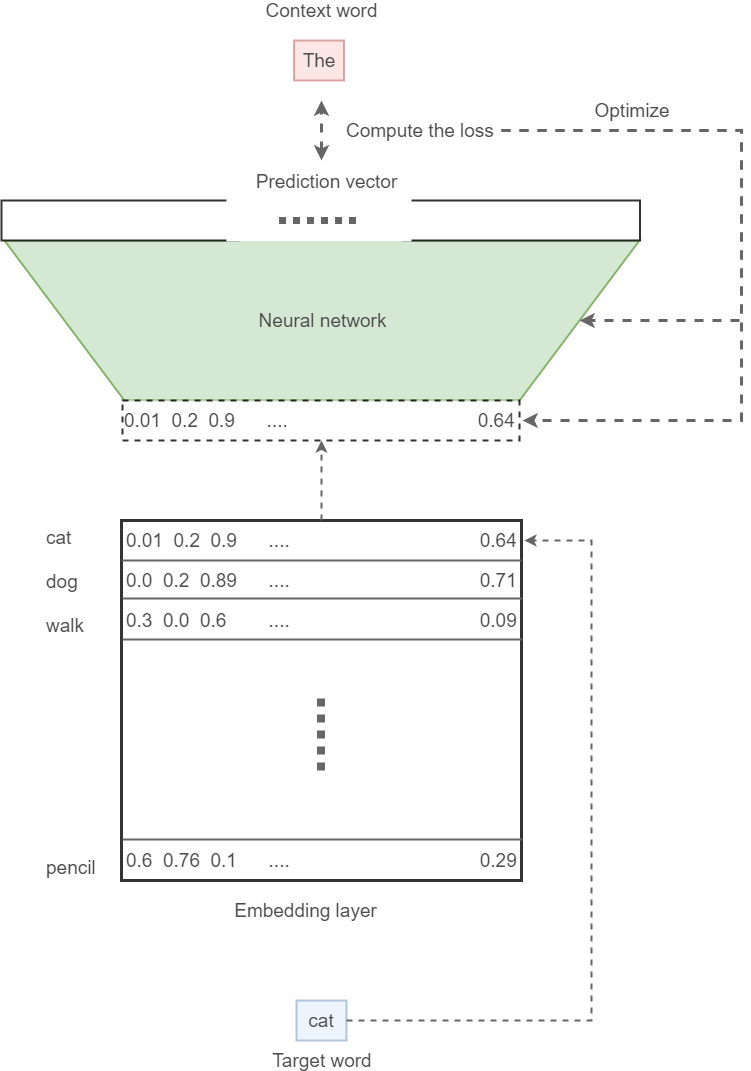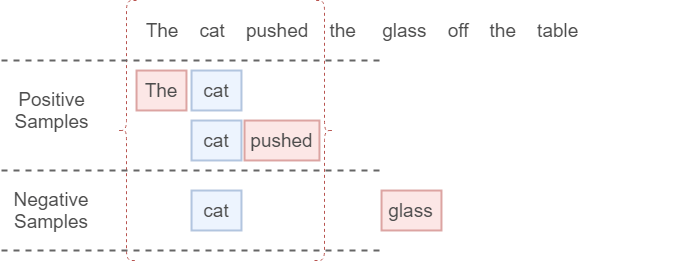2022-05-08
###### Understanding GloVe embedding with Tensorflow implementation
2022-05-10

This article is going to be about Word2vec algorithms. Word2vec algorithms output word vectors. Word vectors, underpin many of the natural language processing (NLP) systems, that have taken the world by a storm (Amazon Alexa, Google translate, etc.). We will talk about the details in the upcoming sections.

## Word vectors, what’s the big idea? It’s all about that context

Without further due, let us stick our feet in. Word vectors are numerical representations of words, that preserve the semantic relationship between words. For example, the vector of the word cat will be very similar to the vector of the word dog. However, the vector for pencil will be quite different from the word vector of cat. And this similarity is defined by the frequency two words in question (i.e. [cat,dog] or [cat,pencil]), are used in the same context. For example, consider the following sentences,

I don’t think I need to spell out the odd one in the above sentences, and obviously the ones with pencil, as the missing word. Why do you feel it as the odd sentence? The spelling is fine, the grammar is right, then why? It is because the context, the word pencil used is not correct. This should convince you of the power the context of a word has, on the word itself. Word vector algorithms use the context of the words to learn numerical representations for words so that words used in the same context have similar-looking word vectors.

## Impact and implications of Word2vec

To get an idea about the implication of the Word2vec techniques, try the following. Go ahead and bring up google scholar. Type in some NLP-related tasks (e.g. question answering, chatbots, machine translation, etc). Filter the papers published after 2013 (that’s when Word2vec methods came out). Get the proportion of the papers using word vectors to the total number of papers. I bet this proportion is going to be quite high. To make the statement concrete, word vectors are used for:

• Language modeling
• Chatbots
• Machine translation
• … and many more

You can see all the exciting NLP frontiers actually depend on word vectors heavily. Now let us discuss what sort of implications word vectors have to make the model better. When using word vectors, semantically close words will appear to be similar computations within the model, whereas other words will appear to do different-looking computations. This is a desirable property to have, as encoding such information in the input itself, leads to the model performing well, with lesser data.

## From raw text to word vectors: high-level approach

Now, with a solid intuition in the bag, we’re going first to discuss the high-level mechanics of the Word2vec algorithms. We’ll polish the details up in later sections until we can be sure that we know how to implement a Word2vec algorithm. In order to learn word vectors in an unsupervised manner (i.e. without human labeling data), we have to define and complete certain tasks. Here’s a high-level list of these tasks.

1. Create data tuples of the format [input word, output word], where each word is represented as one-hot vectors, from raw text
2. Define a model that can take in the one-hot vectors as inputs and outputs, to be trained
3. Define a loss function that predicts the correct word, which is actually in the context of the input word, to optimize the model
4. Evaluate the model by making sure similar words have similar word vectors

This seemingly simple procedure will lead to learning very powerful word vectors. Let us move on to the nitty-gritty of each step of the above pipeline.

# Creating structured data from raw text

This is not a very difficult task. This is a simple manipulation of raw text to put that into a certain structure. Think of the following sentence.

The cat pushed the glass off the table

The data created from this sentence would look as follows. Each row after the sentence represents a single data point. The blue box represents the one-hot input word (the middle word, called the target word), and the red box represents the one-hot output word (any word in the context window except the middle word, called context words). Two data points are created from a single context window. The size of the context window is something defined by the user. Higher the context window size, the better the performance of the model. But when having a large context window size, you’ll pay in computational time, as the amount of data increases. Don’t confuse the target word with the target (correct output) for the neural network, these are two completely different things.

## Defining the embedding layer and the neural network

The neural network is used to learn from the structured data defined above. However, it comes with a twist! To make it clear, you have the following components.

• A batch of inputs represented as one-hot vectors
• A batch of outputs represented as one-hot vectors (only at the training phase)
• An embedding layer
• A neural network

No need to be scared if you did not get a feeling of what and how the last two components perform. We will probe each of these components to understand what they do.

## Embedding layer: stores all the word vectors

First on our agenda is the embedding layer. The embedding layer stores the word vectors of all the words found in the vocabulary. As you can imagine this is an enormous matrix (of size [vocabulary size x embedding size]). This embedding size is a user-tunable parameter. The higher it is, the better performing your model will be. But you’ll not get much of a jaw-dropping performance/size gain, beyond a certain point (say, an embedding size of 500). This gigantic matrix is initialized randomly (just like a neural network) and is tweaked bit by bit, during the optimization process, to reveal the powerful word vectors. This is what it looks like.What the embedding layer looks like

## Neural network: maps word vectors to outputs

Next in line is the last LEGO block of our model; the neural network. During the training, the neural network takes an input word and attempts to predict the output word. Then using a loss function, we penalize the model for incorrect classifications and reward the model for correct classifications. We will be limiting our conversation to processing a single input and a single output at a time. However, in reality, you process data in batches (say, 64 data points). Let’s delineate the exact process used during training:

1. For a given input word (the target word), find the corresponding word vector from the embedding layer
2. Feed the word vector to the neural network, then try to predict the correct output word (a context word)
3. By comparing the prediction and true context word, compute the loss
4. Use the loss along with a stochastic optimizer to optimize the neural network and the embedding layer

One thing to note is, that when computing the prediction, we use a softmax activation to normalize the predictions to a valid probability distribution.

## Fitting all together: inputs to model to outputs

Knowing all the nuts and bolts of the Word2vec algorithm, we can put all the parts together. So that once this model is trained, all we have to do is save the embedding layer to the disk. Then we can enjoy semantic preserved word vectors at any time of the day. Below we see what the full picture looks like.How the model looks in its final form

This particular arrangement of data and the model layout is known as the skip-gram algorithm; a Word2vec algorithm. And this is what we’re going to focus on. The other algorithm is called a continuous bag-of-words (CBOW) model.

## Defining a loss function: to optimize the model

One crucial bit of information we haven’t discussed so far but is essential is the loss function. Normally, standard softmax cross-entropy loss is a good loss function for a classification task. Using this loss is not very practical for a Word2vec model, as for a simpler task like sentiment analysis (where you have 2 possible outputs: positive or negative). Here things can get funky. In a real-world task, that consumes billions of words, the vocabulary size can grow up to 100,000 or beyond easily. This makes the computation of the softmax normalization heavy. This is because the full computation of softmax requires calculating cross-entropy loss with respect to all the output nodes.

So we are going with a smarter alternative, called sampled softmax loss. In sampled softmax loss, you do the following. Note that there are quite a lot of changes from the standard softmax cross-entropy loss. First, you compute the cross-entropy loss between the true context word ID for a given target word and the prediction value corresponding to the true context word ID. Then to that, we add the cross-entropy loss of `K` negative samples we sampled according to some noise distribution. On a high level, we define the loss as follows:

The `SigmoidCrossEntropy` is a loss we can define on a single output node, independent of the rest of the nodes. This makes it ideal for our problem, as our vocabulary can grow quite large. I’m not going to dive into the very details of this loss. You don’t need to understand how exactly this is implemented, as these are available as a built-in function in TensorFlow. But understanding the parameters involved in the loss (e.g. K) is important. The takeaway message is that the sampled softmax loss computes the loss by considering two types of entities:

• The index is given by the true context word ID in the prediction vector (words within the context window)
• `K` indices that indicate word IDs, and are considered to be noise (words outside the context window)

I further visualize this by illustrating an example.Getting positive and negative samples for the sampled softmax layer

# TensorFlow implementation: Skip-gram algorithm

Here we will regurgitate what we just discussed into an implementation. This is available as an exercise here. In this section, we’re going to implement the following.

• A data generator
• The skip-gram model (with TensorFlow)
• Running the skip-gram algorithm

### Data generator

First, let us understand how to generate data. We are not going to go into details of this code, as we have already discussed the internal mechanics of the data generation. This is just converting that logic to an implementation.

```def generate_batch(batch_size, window_size):
global data_index

# two numpy arras to hold target words (batch)
# and context words (labels)
batch = np.ndarray(shape=(batch_size), dtype=np.int32)
labels = np.ndarray(shape=(batch_size, 1), dtype=np.int32)

# span defines the total window size
span = 2 * window_size + 1

# The buffer holds the data contained within the span
queue = collections.deque(maxlen=span)

# Fill the buffer and update the data_index
for _ in range(span):
queue.append(data[data_index])
data_index = (data_index + 1) % len(data)

for i in range(batch_size // (2*window_size)):
k=0
# Avoid the target word itself as a prediction
for j in list(range(window_size))+list(range(window_size+1,2*window_size+1)):
batch[i * (2*window_size) + k] = queue[window_size]
labels[i * (2*window_size) + k, 0] = queue[j]
k += 1

# Everytime we read num_samples data points, update the queue
queue.append(data[data_index])

# If end is reached, circle back to the beginning
data_index = (data_index + np.random.randint(window_size)) % len(data)

return batch, labels
```

### Defining the skip-gram model

First, we will define some hyperparameters required for the model.

```batch_size = 128
embedding_size = 64
window_size = 4
num_sampled = 32 # Number of negative examples to sample.
```

The `batch_size` defines the number of data points we process at a given time. Then the `embedding_size` is the size of a word vector. The next hyperparameter `window_size` defines the size of the context window we visualized above. Finally `num_sampled` defines the number of negative samples in the loss function (`K`). Then we define TensorFlow placeholders for inputs and outputs.

```tf.reset_default_graph()
# Training input data (target word IDs).
train_dataset = tf.placeholder(tf.int32, shape=[batch_size]) # Training input label data (context word IDs)
train_labels = tf.placeholder(tf.int32, shape=[batch_size, 1])
```

Here the `train_dataset` takes a list of word IDs of `batch_size` that represents a selected set of target words. Finally, `train_labels` represents a `batch_size` list of corresponding context words for the selected target words. Next, we define the model parameters required to define the model: the embedding layer and the weights and biases of the neural network.

```################################################
#            Model variables                   #
################################################

# Embedding layer
embeddings = tf.Variable(tf.random_uniform([vocabulary_size, embedding_size], -1.0, 1.0))

# Neural network weights and biases
softmax_weights = tf.Variable(
tf.truncated_normal([vocabulary_size, embedding_size],
stddev=0.1 / math.sqrt(embedding_size))
)
softmax_biases = tf.Variable(tf.random_uniform([vocabulary_size], -0.01, 0.01))
```

We have defined the embedding layer as a TensorFlow variable: `embeddings`. Then we define the neural network weights (`softmax_weights`) and biases (`softmax_biases`). Thereafter we define a key operation required to connect the embedding layer to the neural network to jointly optimize the embedding layer and the neural network.

```# Look up embeddings for a batch of inputs.
embed = tf.nn.embedding_lookup(embeddings, train_dataset)
```

The `tf.nn.embedding_lookup` function takes our embedding layer as the input and a set of word IDs (`train_dataset`) and outputs the corresponding word vectors to the variable `embed`. With the embedding lookup function defined, we can define the sampled softmax loss function we discussed above.

```################################################
#              Computes loss                   #
################################################
loss = tf.reduce_mean(tf.nn.sampled_softmax_loss(weights=softmax_weights, biases=softmax_biases, inputs=embed, labels=train_labels, num_sampled=num_sampled, num_classes=vocabulary_size) )
```

Here the `tf.nn.sampled_softmax_loss` function takes in a set of weights (`softmax_weights`), biases (`softmax_biases`), a set of word vectors corresponding to the word IDs found in `train_dataset`, IDs of the correct context words (`train_labels`), number of noise samples (`num_sampled`) and the size of the vocabulary (`vocabulary_size`). With the output calculation operations and the loss defined, we can define an optimizer to optimize the loss with respect to the parameters of the embeddings layer and the neural network.

```################################################
#                 Optimization                 #
################################################
```

Then we get the normalized embedding layer by making the vector magnitude equal to 1.

```##########                For evaluation                ##########
norm = tf.sqrt(tf.reduce_sum(tf.square(embeddings), 1, keepdims=True))
normalized_embeddings = embeddings / norm
```

### Running the code

Here we are going to discuss the details on how to run the previously defined TensorFlow model. First, we define a `session` and then initialize all the TensorFlow variables randomly.

```num_steps = 250001
session = tf.InteractiveSession()
# Initialize the variables in the graph
tf.global_variables_initializer().run()
print('Initialized')
average_loss = 0
```

Now for a pre-defined number of steps, we generate batches of data: target words (`batch_data`) and context words (`batch_labels`).

```for step in range(num_steps):
# Generate a single batch of data
batch_data, batch_labels = generate_batch(batch_size, window_size)
```

Then for each generated batch, we optimize the embedding layer and the neural network by running `session.run([optimize, loss],...)`. We also get the resulting loss out, to make sure it is decreasing over time.

```    # Optimize the embedding layer and neural network
# compute loss
feed_dict = {train_dataset:batch_data, train_labels:batch_labels}
_, l = session.run([optimizer, loss], feed_dict=feed_dict)
```

Here, every 5000 steps, we print the average loss, as a visual aid.

```    if (step+1) % 5000 == 0:
if step > 0:
average_loss = average_loss / 5000

print('Average loss at step %d: %f' % (step+1, average_loss))
average_loss = 0
```

Finally, we get the final embeddings out, which we later use for visualization of certain words.

```sg_embeddings = normalized_embeddings.eval()
session.close()
```

Finally, if you visualize the embeddings using a manifold learning algorithm like t-SNE, you will get the following.

As you can see the words related to cat are found in a certain direction, and words related to the dog are found in a different direction. And words that fall between (e.g. animal or pet) fall between these two directions, which is pretty much what we needed.

## Conclusion

This brings us to the end of our conversation. Word vectors are a very powerful representation of words that helps the machine learning models to perform better. We went through the data generation process as well as different components found in a Word2vec model. Then we discussed one specific variant of a Word2vec algorithm; a skip-gram model. We went through the implementation of the algorithm in TensorFlow. Finally, we visualized the embeddings and saw that the learned embeddings actually depict some useful semantics. You can find the exercise file here.

Source:

https://towardsdatascience.com/light-on-math-machine-learning-intuitive-guide-to-understanding-word2vec-e0128a460f0f##### Amir Masoud Sefidian
Machine Learning Engineer